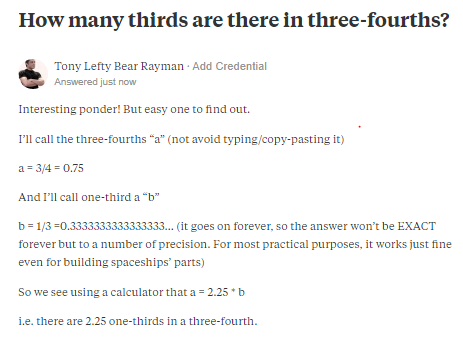Friday, September 22, 2023

# How many thirds in a 3-quarter?

Recently Quora notified me with this question and I thought I’d do the honor of answering it first. So here’s the answer and explanation. Now, how about a different way solving it?Put another way, we can pose the problem as 1/3x = 3/4 and solve for x.

If 1/3 = 0.333 (approx.)

Then the above equation to solve for x is: x = 3/(4*0.333) = 2.25